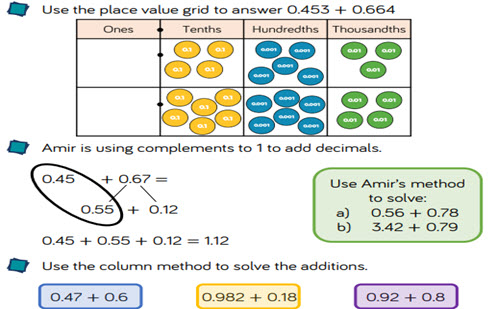Please note: This site contains links to websites not controlled by the Australian Government or ESA. More information here.

## Decimals: operating with decimals

This guide provides a sequence of small steps for learning to operate with decimals, from adding and subtracting decimals through to multiplying and dividing decimals by 10, 100 and 1,000.

Year level(s) Year 6
Audience Teacher
Purpose Teaching resource, Teaching strategies
Teaching strategies and pedagogical approaches Questioning, Concrete Representational Abstract model, Explicit teaching
Keywords decimals, decimal place, adding decimals, subtracting decimals, multiplying decimals, dividing decimals, decimal sequences, ones, tenths, hundredths, thousandths, reasoning, problem-solving, explicit teaching

### Curriculum alignment

Curriculum connections Numeracy
Strand and focus Number, Build understanding
Topics Decimals, Addition and subtraction, Multiplication and division, Place value
AC: Mathematics content descriptions
ACMNA128 Add and subtract decimals, with and without digital technologies, and use estimation and rounding to check the reasonableness of answers
ACMNA130 Multiply and divide decimals by powers of 10
National numeracy learning progression Operating with decimals - OwD1, OwD2, OwD3